1. Home >
2. Apps >
3. Groups >

# What is the relationship between frequency and distance?

Question asked by Olu_Ola in #Electrical #Electronics on Sep 6, 2013Olu_Ola · Sep 6, 2013
Rank E1 - BEGINNER

Please can anybody explain how the frequency of a communication signal affect its propagation range?

Posted in: #Electrical #Electronics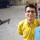Abhishek Rawal · Sep 6, 2013
Rank A2 - PRO
Low frequency(LF) is longer than High Frequency (HF) signals, hence it has less penetration power. So, when it comes to sending information signals to larger distance, LF fails. (Which means LF can carry less information)
While HF has high penetration power, thus it can easily send information to large distance. (Which means HF carries more information)
Also Power plays major role in information transmission. larger the power, larger the distance covered.lal · Sep 6, 2013
Rank A3 - PRO
I thought it was the other way around. Low frequency signals are of larger wavelength than that of high frequency waves and they travel longer, for the same power.

In the case of visible light, waves of shorter wavelength/high frequency travels less (blue light) and those with larger wavelength/low frequency travels longer (red light) distances.

Isn't it the case with coomunication signals too. Besides those are all electromagnetic waves.Abhishek Rawal · Sep 6, 2013
Rank A2 - PRO
E = hf
Which means Energy is directly proportional to frequency.
Low frequency have low energy, so less distance traveled & thus HF can travel long distance.

Correct me if I am wrong.Jeffrey Arulraj · Sep 6, 2013
Rank A2 - PRO
E=h*V

h= planck's constant
v= frequency of signal

So higher the frequency longer the distance you can transfer

Most of all this is not a good way to look into communication do tell in What form you are trying to transfer data

Digital or Analog format ?Abhishek Rawal · Sep 6, 2013
Rank A2 - PRO
Jeffrey Samuel
Digital or Analog format ?
I think, Digital signals cannot be transmitted between two antennas (i.e through air)Olu_Ola · Sep 6, 2013
Rank E1 - BEGINNER
Thanks All, but is there a mathematical equation that can approximate the maximum distance a signal will travel in a free space before it becomes too weak to be received by any antenna, given a specific frquency (say 2.4GHz)?.

I use free space bearing in mind that so many factors affect signal quality in our atmosphere.Jeffrey Arulraj · Sep 7, 2013
Rank A2 - PRO
Can you share the equation or the name of the equation you found?

@Olu_OlaAhsanul haque · Sep 16, 2013
Rank C3 - EXPERT
Frequency has nothing to do with distance, the only reason frequency is sometimes associated with distance, is the length of one full RF cycle in the air, this is calculated with the following formula:
speed= distance /time
speed=wavelength*frequency So, wavelength*frequency=distance/time
And, wavelength=distance/(time*frequency)
Frequency and distance are directly proportional the higher the frequency the more distance for the sound to dissipate th lower the frequency th shorter the distance for the sound to dissipate
ThanksDarren Simpson · Apr 8, 2015
Rank E2 - BEGINNER
The communications signal distance travelled is based upon the total link loss available in the system. If the tx is +40dBm and Rx sensitivity is -100dBm then the total link loss available is 140dB. In a free space environment, lower frequencies will travel further over higher frequencies (K+20logd+20logf where K is the Boltzmann constant d is distance and f is frequency).

VHF 120MHz is great for long distance such as ground to air comms at an airport and can talk to planes miles away. UHF 450MHz is still good for distance but not as far and is good for penetration into buildings over VHF due to its smaller wavelength size compared to the size of construction materials.Alphin · Jun 15, 2017
Rank E1 - BEGINNER
Frequency actually has various characteristics ..for sky wave n ground wave..in general high frequency has lower wave length so it disturbed due to more vibration...but low frequency has greater wavelength so it is not disturbed easily by molecules..so low frequency can travel further..but many asked RF transmission is high frequency..how does it travel further.. actually high frequency can carry more information .when it is transmitted with higher power even high frequency can travel longer....to be clear ..consider same frequency with different power ..the number of vibration will be same only..but the amplitude will increase and the envelope will be big..which makes it easier to travel longer.....Alphin · Aug 27, 2017
Rank E1 - BEGINNER
Abhishek Rawal
E = hf
Which means Energy is directly proportional to frequency.
Low frequency have low energy, so less distance traveled & thus HF can travel long distance.

Correct me if I am wrong.Alphin · Aug 27, 2017
Rank E1 - BEGINNER
Iam an electronics n communication engineer..people have misunderstood the concept wrongly...low frequency signals have larger wavelength ..so it's vibration is less so it is easily not disturbed ...it can travel long distance..high frequency has higher vibration n lower wavelength..it cannot travel long...for example consider microwave em wave since it's wavelength is low it can break the bond of water molecule ...if it's wavelength is high it will neglect several molecules n break few bonds ..it is how microwave oven works..so higher the frequency higher the disturbance n lower the propogation..I have undergone internship at air navigation n surveillance..CNS ..UHF transmitters are used for short distance..hf transmitter for long distance....there was an answer stating that E=hf ..I agree energy increases ..but that energy doesn't mean that it can travel longer..it means it can disturb more..that's why microwave frequency produces heat ..but people my wonder how microwave is used in ovens n also in satellite communications...... microwave is high frequency ..so it has many cycles per second...so in sattelite communication data rate can be transferred high...but now comes the doubt..how high frequency can be transmitted so far...now POWER plays an important role...when power is increased voltage is increased the wavelet becomes larger ..but it vibrates in same frequency only..same frequency with different power travel different distance..as power is related to volt or amplitude of the wave..so wavelet becomes larger ...high data rate using mw frequency ..at the same time with high power.....Sry if this answer was too lengthyLouis Chung · Oct 23, 2018
Rank E1 - BEGINNER

Total energy =n* hf

If we apply the same power for high and low frequency wave, the number of photon would be inversely proportional to the frequency. Thus more photons will increase the tranvel range  im air at lower frequencyJ Lee Hughes · Dec 14, 2018
Rank E1 - BEGINNER

High hz more data

But there is a curve rely low go sHort but ground esh middle bonus of atmosphere and really high frequencies can go out to space

When you get into really high frequencies becomes more line of sight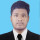Akash Pswamy · Jan 18, 2020
Rank D3 - MASTER

First of all we need to understand the relation between the wavelength and frequency telling fw=c where f is the frequency, c is the speed of light, and w is the wavelength. f and w are inversely related. Let us see the order of arrangement of the EM waves such as starting from gamma waves, xray, infrared, visible, microwave amd radiowaves( sorry if i have missed any). And let us consider the visible band of the EM waves by which we all see the red light has the longest travel distance because its wavelength is the highest among all the visible colours. The famous example of the traffic light because of which red light was considered so that it would be seen to a longer distance so people stop the vehicle in time. Keeping this in mind the wavelength is highest for red and lowest for violet in the visible band and is arranged in the order red, orange, yellow, green, blue, violet( leaving out the intermediate colours).

So by this patter we see that to the right of visible band the wavelength decreases and to the left the wavelength increases. Meaning the X-ray has a better penetration ability as its frequency is low and wavelength is high. And gamma rays has the most penetrating ability of all as for an example X-ray is used to penetrate bones where as microwave and radio waves used in radar us to penetrate clouds. So the pentration ability increases with increase in wavelength which inturn means decrease of frequency helps in increase of penetration ability of a wave. And hence we can tell that the propagation range is highest for the wave with highest wavelength and lowest frequency.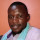bill yorke · Jan 19, 2020
Rank C2 - EXPERT

based on dimensional analysis, relationship between wavelength and distance relates to one shared between frequency and duration but not any of the pair is similar. While frequency is cycles divided by time, wavelength is a derivative of distances divided by cycles.  when the two are multiplies the cycles cancel out from which you get velocity, that is  distance divided by time.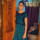Kavya Burramolla · Jan 20, 2020
Rank C1 - EXPERT

Firstly, Wavelength and frequency are two different properties of the signal. When a signal is propagated through a wire or free space, wavelength corresponds to the distance between to identical points(two identical points can be peak to peak points or any other similar points) in the adjacent cycles of the waveform.

Whereas the frequency of the signal is defined as the number of times one cycle of waveform is repeated per second. We know that velocity of a wave(v)= distance travelled by the wave(d) over a period of time(t). That is v = d/t. Here distance between two identical points in the wave is given by wavelength and frequency is inverse of time. Hence, the speed of light(c) is given by the product of frequency and wavelength.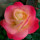supriya VN gowda · Feb 26, 2020
Rank C2 - EXPERT

Frequency is number of waves that pass through a given area at fixed time. It's unit is hertz.

Distance is the measurement of how far apart objects are. It's unit will be kilometer.

Frequency of sound is increased or decreased based on the distance between the objects. These are inversely propotional.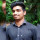Teja Reddy · Feb 27, 2020
Rank C3 - EXPERT

The relationship between wavelength and distance is similar to the relationship between frequency and duration, and no: neither pair is the same. You can see by using dimensional analysis. Wavelength is distance divided by cycles. Frequency is cycles divided by time.

## You must log-in or sign-up to reply to this post.

Click to Log-In or Sign-Up